JEE  >  JEE Main Previous year questions (2016-22): Classification of Elements & Periodicity in Properties

# JEE Main Previous year questions (2016-22): Classification of Elements & Periodicity in Properties Notes | Study Chemistry for JEE - JEE

## Document Description: JEE Main Previous year questions (2016-22): Classification of Elements & Periodicity in Properties for JEE 2022 is part of Chemistry for JEE preparation. The notes and questions for JEE Main Previous year questions (2016-22): Classification of Elements & Periodicity in Properties have been prepared according to the JEE exam syllabus. Information about JEE Main Previous year questions (2016-22): Classification of Elements & Periodicity in Properties covers topics like and JEE Main Previous year questions (2016-22): Classification of Elements & Periodicity in Properties Example, for JEE 2022 Exam. Find important definitions, questions, notes, meanings, examples, exercises and tests below for JEE Main Previous year questions (2016-22): Classification of Elements & Periodicity in Properties.

Introduction of JEE Main Previous year questions (2016-22): Classification of Elements & Periodicity in Properties in English is available as part of our Chemistry for JEE for JEE & JEE Main Previous year questions (2016-22): Classification of Elements & Periodicity in Properties in Hindi for Chemistry for JEE course. Download more important topics related with notes, lectures and mock test series for JEE Exam by signing up for free. JEE: JEE Main Previous year questions (2016-22): Classification of Elements & Periodicity in Properties Notes | Study Chemistry for JEE - JEE
 1 Crore+ students have signed up on EduRev. Have you?

Q.1. The first ionization enthalpy of Na, Mg and Si, respectively, are : 496, 737 and 786 kJ mol−1. The first ionization enthalpy (kJmol−1) of Al is :             (JEE Main 2022)
(a) 487
(b) 768
(c) 577
(d) 856

Ans. c
I.E. Na<Al<Mg<Si
∵496< I.E. (Al) <737
Option (C), matches the condition
i.e. I.E. (Al) = 577 kJ mol-1

Q.2. In which of the following pairs, electron gain enthalpies of constituent elements are nearly the same or identical?             (JEE Main 2022)
(A) Rb and Cs
(B) Na and K
(C) Ar and Kr
(D) I and At
Choose the correct answer from the options given below :             (JEE Main 2022)
(a) (A) and (B) only
(b) (B) and (C) only
(c) (A) and (C) only
(d) (C) and (D) only

Ans. c
Rb & Cs have nearly same electron gain enthalpy electron gain enthalpy = −46kj/ml
Ar & Kr have same ΔHeq. Value is + 96 kj/ml

Q.3. The incorrect statement is             (JEE Main 2022)
(a) The first ionization enthalpy of K is less than that of Na and Li.
(b) Xe does not have the lowest first ionization enthalpy in its group.
(c) The first ionization enthalpy of element with atomic number 37 is lower than that of the element with atomic number 38.
(d) The first ionization enthalpy of Ga is higher than that of the d-block element with atomic number 30.

Ans. d
On moving down in a group ionisation energy decrease
∴1st  ionisation enthalpy order is Li>Na>K
Zn has more ionisation energy as compared to Ga because of their pseudo inert gas configuration.

Q.4. Given two statements below :             (JEE Main 2022)
Statement I : In Cl2 molecule the covalent radius is double of the atomic radius of chlorine.
Statement II : Radius of anionic species is always greater than their parent atomic radius.
Choose the most appropriate answer from options given below :

(a) Both Statement I and Statement II are correct.
(b) Both Statement I and Statement II are incorrect.
(c) Statement I is correct but Statement II is incorrect.
(d) Statement I is incorrect but Statement II is correct.

Ans. d
Covalent radius is not double of atomic radius.
Radius of anionic species is always greater than their parent atomic radius as nuclear charge decreases in anionic counterpart.

Q.5. The first ionization enthalpies of Be, B, N and O follow the order             (JEE Main 2022)
(a) O < N < B < Be
(b) Be < B < N < O
(c) B < Be < N < O
(d) B < Be < O < N

Ans. d
The first ionization energy increase from left to right along 2nd  period with the following exceptions
IE1 : Be > B and N > O
This is due to stable configuration of Be in comparison to B and that of N in comparison to O.
Hence the correct order is N > O > Be > B

Q.6. The IUPAC nomenclature of an element with electronic configuration [Rn] 5f146d17s2 is :             (JEE Main 2022)
(a) Unnilbium
(b) Unnilunium
(d) Unniltrium

Ans. d
The element with electronic configuration [Rn] 5f146 d17 s2 has atomic number → 103
∴ Its IUPAC name is: Unniltrium

Q.7. The correct order of electron gain enthalpy (− ve value) is :             (JEE Main 2022)
(a) O > S > Se > Te
(b) O < S < Se < Te
(c) O < S > Se > Te
(d) O < S > Se < Te

Ans. c

Q.8. The correct order of electron gain enthalpies of Cl, F, Te and Po is             (JEE Main 2022)
(a) F < Cl < Te < Po
(b) Po < Te < F < Cl
(c) Te < Po < Cl < F
(d) Cl < F < Te < Po

Ans. b
Te → –190 kJ mol–1
Po → –174 kJ mol–1
F → –333 kJ mol–1
Cl → –349 kJ mol–1
Hence, correct order is Cl > F > Te > Po

Q.9. Given below are two statements : one is labelled as Assertion (A) and the other is labelled as Reason (R).             (JEE Main 2022)
Assertion (A) : The ionic radii of O2− and Mg2+ are same.
Reason (R) : Both O2− and Mg2+ are isoelectronic species.
In the light of the above statements, choose the correct answer from the options given below.
(a) Both (A) and (R) are true and (R) is the correct explanation of (A).
(b) Both (A) and (R) are true but (R) is not the correct explanation of (A).
(c) (A) is true but (R) is false.
(d) (A) is false but (R) is true.

Ans. a
Correct order of ionic radii:
O–2 > Mg+2
This is because among isoelectronic species, the size of anions are greater than the size of cations. Statement (II) is correct as both O–2 and Mg+2 are isoelectronic.

Q.10. The correct order of increasing ionic radii is             (JEE Main 2022)
(a) Mg2+ < Na+ < F− < O2− < N3−
(b) N3− < O2− < F < Na+ < Mg2+
(c) F < Na+ < O2− < Mg2+ < N3−
(d) Na+ < F < Mg2+ < O2− < N3−

Ans. a
For isoelectronic species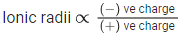Hence, correct order of ionic radii is
Mg2+ < Na+ < F < O2– < N3–

Q.11. Element "E" belongs to the period 4 and group 16 of the periodic table. The valence shell electron configuration of the element, which is just above "E" in the group is             (JEE Main 2022)
(a) 3s2, 3p4
(b) 3d10, 4s2, 4p4
(c) 4d10, 5s2, 5p4
(d) 2s2, 2p4

Ans. a
Element E is Selenium (Se)
Electronic configuration of E is [Ar] 3d10 4s2 4p4
The element which is just above ‘E’ in periodic table is sulphur, its electronic configuration is [Ne] 3s2 3p4

Q.12.  Given below are two statements. One is labelled as Assertion A and the other is labelled as Reason R.             (JEE Main 2022)
Assertion A : The first ionization enthalpy for oxygen is lower than that of nitrogen.
Reason R : The four electrons in 2p orbitals of oxygen experience more electron-electron repulsion.
In the light of the above statements, choose the correct answer from the options given below.
(a) Both A and R are are correct and R is the correct explanation of A.
(b) Both A and R are are correct but R is NOT the correct explanation of A.
(c) A is correct but R is not correct.
(d) A is not correct but R is correct.

Ans. b
Nitrogen has half-filled p-orbitals which is stable. Due to this, its 1st ionization energy is more than oxygen.

Q.13. The electronic configuration of Pt (atomic number 78) is:             (JEE Main 2022)
(a) [Xe] 4f14 5d9 6s1
(b) [Kr] 4f14 5d10
(c) [Xe] 4f14 5d10
(d) [Xe] 4f14 5d8 6s2

Ans. a
Atomic number of Pt is 78
Electronic configuration is – 78Pt → [Xe] 4 f14 5d9 6s1 (Exceptional electronic configuration)

Q.14. Identify the element for which electronic configuration in +3 oxidation state is [Ar]3d5 :             (JEE Main 2021)
(a) Ru
(b) Mn
(c) Co
(d) Fe

Ans. d
Mn(25) = [Ar]3d54s2
Mn+3 = [Ar]3d44s0
Ru-belongs to 4d transition series
Co (27) = [Ar]3d74s2
Co+3 = [Ar]3d64s0
Fe(26) = [Ar]3d64s2
Fe+3 = [Ar]3d54s0

Q.15. Match List - I with List - II :              (JEE Main 2021)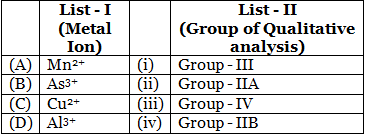Choose the most appropriate answer from the options given below :
(a) (A)-(i), (B)-(ii), (C)-(iii), (D)-(iv)
(b) (A)-(iii), (B)-(iv), (C)-(ii), (D)-(i)
(c) (A)-(i), (B)-(iv), (C)-(ii), (D)-(iii)
(d) (A)-(iv), (B)-(ii), (C)-(iii), (D)-(i)

Ans. b
Mn2+ → III group
As3+ → II B group
Cu2+ → II A group
Al3+ → IV group

Q.16. The Eu2+ ion is a strong reducing agent in spite of its ground state electronic configuration (outermost) : [Atomic number of Eu = 63]              (JEE Main 2021)
(a) 4f76s2
(b) 4f6
(c) 4f7
(d) 4f66s2

Ans. c
Eu → [Xe]4f76s2
Eu2+ → [Xe]4f7

Q.17. Given below are two statements : one is labelled as Assertion (A) and the other is labelled as Reason (R).              (JEE Main 2021)
Assertion (A) : Metallic character decreases and non-metallic character increases on moving from left to right in a period.
Reason (R) : It is due to increase in ionisation enthalpy and decrease in electron gain enthalpy, when one moves from left to right in a period.
In the light of the above statements, choose the most appropriate answer from the options given below :

(a) (A) is false but (R) is true.
(b) (A) is true but (R) is false
(c) Both (A) and (R) are correct and (R) is the correct explanation of (A)

(d) Both (A) and (R) are correct but (R) is not the correct explanation of (A)

Ans. b
From left to right in periodic table:-
Metallic character decreases
Non-metallic character increases
⇒ It is due to increase in ionization enthalpy and increase in electron gain enthalpy.

Q.18. Which one of the following lanthanides exhibits +2 oxidation state with diamagnetic nature ? (Given Z for Nd = 60, Yb = 70, La = 57, Ce = 58)              (JEE Main 2021)
(a) Nd
(b) Yb
(c) La
(d) Ce

Ans. b
Yb (70) = 4f14 6s2
Yb+2 = 4f14 6s0
∵ All the electrons are paired hence Yb+2 is diamagnetic.

Q.19. Which one of the following is used to remove most of plutonium from spent nuclear fuel?              (JEE Main 2021)
(a) ClF3
(b) O2F2
(c) I2O5
(d) BrO3

Ans. b
O2F2 oxidizes plutonium to PuF6 and the reaction is used in removing plutonium as PuF6 from spent nuclear fuel.

Q.20. The correct order of ionic radii for the ions, P3−, S2−, Ca2+, K+, Cl is :               (JEE Main 2021)
(a) P3− > S2− >Cl > K+ > Ca2+
(b) Cl > S2− > P3− > Ca2+ > K+
(c) P3− > S2− > Cl > Ca2+ > K+
(d) K+ > Ca2+ > P3− > S2− > Cl

Ans. a
P3− > S2− > Cl > K+ > Ca2+ (Correct order of ionic radii)
all the given species are isoelectronic species. In isoelectronic species size increases with increase of negative charge and size decreases with increase in positive charge.

Q.21. Choose the correct statement from the following :              (JEE Main 2021)
(a) The standard enthalpy of formation for alkali metal bromides becomes less negative on descending the group.
(b) The low solubility of CsI in water is due to its high lattice enthalpy.
(c) Among the alkali metal halides, LiF is least soluble in water.
(d) LiF has least negative standard enthalpy of formation among alkali metal fluorides.

Ans. c
a. Standard enthalpy of formation for alkali metal bromides becomes more negative on descending down the group.
b. In case of CsI, lattice energy is less, but Cs+ is having less hydration enthalpy due to which it is less soluble in water.
c. For alkali metal fluorides, the solubility in water increases from lithium to caesium. LiF is least soluble in water.
d. Standard enthalpy of formation for LiF is most negative among alkali metal fluorides.

Q.22. In which one of the following molecules strongest back donation of an electron pair from halide to boron is expected?              (JEE Main 2021)
(a) BCl3
(b) BF3
(c) BBr3
(d) BI3

Ans. b
Type of back bonding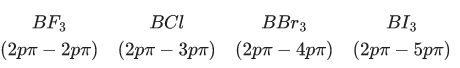Therefore back bonding strength is as follows
BF3 > BCl > BBr3 > BI3

Q.23. Chalcogen group elements are :              (JEE Main 2021)
(a) Se, Tb and Pu.
(b) Se, Te and Po.
(c) S, Te and Pm.
(d) O, Ti and Po.

Ans. b
Group 16/oxygen family is known as Chalcogens the members are O, S, Se, Te, Po

Q.24. Given below are two statements : one is labelled as Assertion (A) and the other is labelled as Reason (R).              (JEE Main 2021)
Assertion (A) : Barium carbonate is insoluble in water and is highly stable.
Reason (R) : The thermal stability of the carbonates increases with increasing cationic size.

(a) Both (A) and (R) are true and (R) is the true explanation of (A)
(b) (A) is true but (R) is false
(c) Both (A) and (R) are true but (R) is not the true explanation of (A)
(d) (A) is false but (R) is true.

Ans. a
In IIA group on moving down the group size of cation increases and show thermal stability of carbonate increases.

Q.25. The correct order of first ionisation enthalpy is :              (JEE Main 2021)
(a) Mg < S < Al < P
(b) Mg < Al < S < P
(c) Al < Mg < S < P
(d) Mg < Al < P < S

Ans. c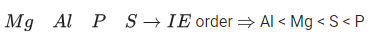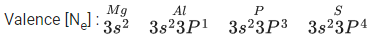Q.26. The ionic radii of F and O2− respectively are 1.33Å and 1.4Å, while the covalent radius of N is 0.74Å.              (JEE Main 2021)
The correct statement for the ionic radius of N3− from the following is :
(a) It is smaller than F− and N
(b) It is bigger than O2− and F
(c) It is bigger than F and N, but smaller than of O2−
(d) It is smaller than O2− and F, but bigger than of N

Ans. b
F, O2− and N3− all are isoelectronic species in which N3− have least number of protons due to which it's size increases as least nuclear attraction is experienced by the outer shell electrons. Size order
N3− > O2− > F

Q.27. The ionic radii of K+, Na+, Al3+ and Mg2+ are in the order:               (JEE Main 2021)
(a) Na+ < K+ < Mg2+ < Al3+
(b) Al3+ < Mg2+ < K+ < Na+
(c) Al3+ < Mg2+ < Na+ < K+
(d) K+ < Al3+ < Mg2+ < Na+

Ans. c
Al3+, Mg2+ and Na+ are isoelectronic ionic species. For monoatomic ionic isoelectronic species as positive charge increases ionic size decreases.
The order of size of Na+ & K+ is Na+ < K+
∴ Order of ionic radii is : Al3+ < Mg2+ < Na+ < K+

Q.28. The set having ions which are coloured and paramagnetic both is -              (JEE Main 2021)
(a) Cu2+, Cr3+, Sc+
(b) Cu2+, Zn2+, Mn4+
(c) Sc3+, V5+, Ti4+
(d) Ni2+, Mn7+, Hg2+

Ans. a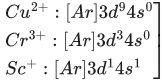All are coloured and paramagnetic due to presence of unpaired electrons

Q.29. Which one of the following statements for D.I. Mendeleeff, is incorrect?              (JEE Main 2021)
(a) He authored the textbook - Principles of Chemistry
(b) At the time, he proposed Periodic Table of elements structure of atom was known.
(c) Element with atomic number 101 is named after him.
(d) He invented accurate barometer.

Ans. b
In 1869, the Russian chemist Dmitri Mendeleev came to prominence with his tabular diagram of known elements, but basic atomic structure the idea that everything is made of atoms was pioneered by John Dalton (1766-1844) in a book he published in 1808.

Q.30. The first ionization energy of magnesium is smaller as compared to that of elements X and Y, but higher than that of Z. The elements X, Y and Z, respectively, are :              (JEE Main 2021)
(a) neon, sodium and chlorine
(b) argon, chlorine and sodium
(c) chlorine, lithium and sodium
(d) argon, lithium and sodium

Ans. b
Order of I.E.
3rd period → Na < Al < Mg < Si < S < P < Cl < Ar

Q.31. The common positive oxidation states for an element with atomic number 24, are :              (JEE Main 2021)
(a) +1 and +3
(b) +2 to +6
(c) +1 to +6
(d) +1 and +3 to +6

Ans. b
24Cr = [Ar]3d54s1
Common positive oxidation state of Cr are from +2 to +6
where +3 and +6 are the most common ones.

Q.32. The absolute value of the electron gain enthalpy of halogens satisfies :              (JEE Main 2021)
(a) Cl > Br > F > I
(b) Cl > F > Br > I
(c) I > Br > Cl > F
(d) F > Cl > Br > I

Ans. b
The magnitude of electron gain enthalpy of halogen atoms down the group show abnormal behavior. The |ΔHeg| of F is lower than that of Cl due to its smaller size. The incoming electron experiences higher repulsive force due to valence electrons of F than Cl. The correct order is Cl > F > Br > I.

Q.33. Identify the elements X and Y using the ionisation energy values given below :              (JEE Main 2021)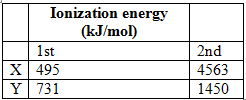(a) X = F; Y = Mg
(b) X = Mg; Y = F
(c) X = Na; Y = Mg
(d) X = Mg; Y = Na

Ans. c
Due to 2p6, noble gas electronic configuration, the second ionisation enthalpy of Na is very high.
That’s why has large difference between IE1, and IE2
Mg+ is 2p6, 3s1.
After the loss of one electron, Mg+ will be formed with noble gas electronic configuration. That’s why has less difference between I.E1 and I.E2, but it's I.E3 is very high.

Q.34. The characteristics of elements X, Y and Z with atomic numbers, respectively, 33, 53 and 83 are :              (JEE Main 2021)
(a) X and Y are metalloids and Z is a metal.
(b) X and Z are non-metals and Y is a metalloid.
(c) X is a metalloid, Y is a non-metal and Z is a metal.
(d) X, Y and Z are metals.

Ans. c
X(Z = 33) = As (metalloid)
Y(Z = 53) = I (non-metal)
Z(Z = 83) = Bi (metal)

Q.35. Given below are two statement : one is labelled as Assertion A and the other is labelled as Reason R :              (JEE Main 2021)
Assertion A : Size of Bk3+ ion is less than Np3+ ion.
Reason R : The above is a consequence of the lanthanoid contraction.
In the light of the above statements, choose the correct answer from the options given below:
(a) Both A and B are true and R is the correct explanation of A
(b) A is false but R is true
(c) A is true but R is false
(d) Both A and B are true but R is not the correct explanation of A

Ans. c
Size of Bk3+ is 98 pm
Size of Np3+ is 101 pm
So size of Np3+ is more than Bk3+ ion.
there is a gradual decrease in the size of M3+ ions across the series. This may be referred to as the actinoid contraction.

Q.36. The correct order of electron gain enthalpy is :              (JEE Main 2021)
(a) Te > Se > S > O
(b) S > Se > Te > O

(c) S > O > Se > Te
(d) O > S > Se > Te

Ans. b
Oxygen is the second most electronegative element in comparison to fluorine. In group - 16 family (O, S, Se, Te), O-atom is smallest in size. So, electron density on O-atom is very high in group -16 During addition of a free electron to gaseous O-atom,
O(g) + e− ⟶ O(g)
We have to supply a significant amount of energy (endothermic) to overcome the electrostatic repulsion between the approaching electron and O-atom of very high electron density. So, the net value of electron affinity (EA) or (negative) electron gain enthalpy [ΔegH or |ΔegH|] of oxygen decreases to a higher extent in comparison to other elements of group -16 who have larger size and lower electronegativity.
So, the correct order of EA or |ΔegH| of group −16 elements will be S > Se > Te > O

Q.37. Match List - I with List - II.              (JEE Main 2021)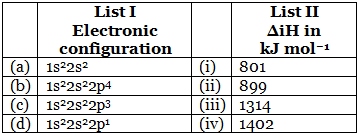Choose the most appropriate answer from the options given below :
(a) (A) → (i), (B) → (iii), (C) → (iv), (D) → (iii)
(b) (A) → (iv), (B) → (i), (C) → (ii), (D) → (iii)
(c) (A) → (ii), (B) → (iii), (C) → (iv), (D) → (i)
(d) (A) → (i), (B) → (iv), (C) → (iii), (D) → (ii)

Ans. c
(a) 1s2 2s2 → Be
(b) 1s2 2s2 2p4 → O
(c) 1s2 2s2 2p3 → N
(d) 1s2 2s2 2p1 → B
The ionization enthalpy order is
B < Be < O < N
Be has more IE compared to B due to extra stability & N has more IE compared to oxygen due to extra stability.
Hence, N → 1402 kJ/mol
O → 1314 kJ/mol
B → 801 kJ/mol
Be → 899 kJ/mol

Q.38. The correct order of bond dissociation enthalpy of halogens is :              (JEE Main 2021)
(a) F2 > Cl2 > Br2 > I2
(b) Cl2 > Br2 > F2 > I2
(c) I2 > Br2 > Cl2 > F2

(d) Cl2 > F2 > Br2 > I2

Ans. b
Among halogens (F2, Cl2, Br2 and I2), bond dissociation enthalpy (ΔdissH) of I2, is minimum because of larger size of I-atom there is a steric repulsion between bonded I-atoms, which makes I-I bond weakest.
Whereas, smaller size and highest electronegativity of F-atom cause highest electron density on F-atom of F2 molecule. As a result, F-F bond becomes weaker due to electrostatic repulsion between bonded F-atoms.
Thus, the order of ΔdissH (in kJ mol−1) is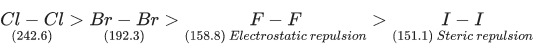Q.39. What is the correct order of the following elements with respect to their density?              (JEE Main 2021)
(a) Cr < Zn < Co < Cu < Fe
(b) Cr < Fe < Co < Cu < Zn
(c) Zn < Cr < Fe < Co < Cu
(d) Zn < Cu < Co < Fe < Cr

Ans. c
Generally, due to decrease in metallic radius and increase in atomic mass density increase across the period from left to right.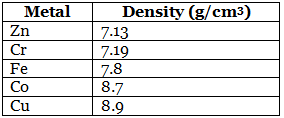Correct order is Cu > Co > Fe > Cr > Zn.

Q.40. Consider the elements Mg, Al, S, P and Si, the correct increasing order of their first ionization enthalpy is :              (JEE Main 2021)
(a) Al < Mg < Si < S < P
(b) Al < Mg < S < Si < P
(c) Mg < Al < Si < S < P
(d) Mg < Al < Si < P < S

Ans. a
On moving left to right in a period of the periodic table, Ionisation energy (I.E.) increases due to increase in effective nuclear charge i.e. Zeff.
But due to extra stability of fully filled and half-filled electronic configuration of Mg and P required ionisation enthalpy is more from neighbouring elements.
i.e. First ionisation enthalpy order is Al < Mg < Si < S < P.

Q.41. Within each pair of elements F and Cl, S and Se, and Li and Na, respectively, the elements that release more energy upon an electron gain are    
(a) Cl, Se and Na
(b) Cl, S and Li
(c) F, S and Li
(d) F, Se and Na

Correct Answer is Option (b)

The electron gain enthalpy of Cl is more than that of F. Thus the pair that is Cl, S and Li releases more energy than F, S and Li. The electron gain enthalpy of S and Li is more than Se and Na respectively.

Q.42. The first ionization energy (in kJ mol–1) of Na, Mg, Al and Si respectively, are    
(a) 496, 737, 577, 786
(b) 496, 577, 737, 786
(c) 786, 737, 577, 496
(d) 496, 577, 786, 737

Correct Answer is Option (a)

Moving from left to right in a period, the size of the element decreases and the value of first ionization enthalpy increases. Thus, the ionization energy of Na, Mg, Al and Si should be 496, 577, 737 and 786 kJ mol–1 respectively but due to the entry of the d-orbital in 3rd period the effective nuclear charge of Al increases. So the ionization energy of Al is lower than Mg.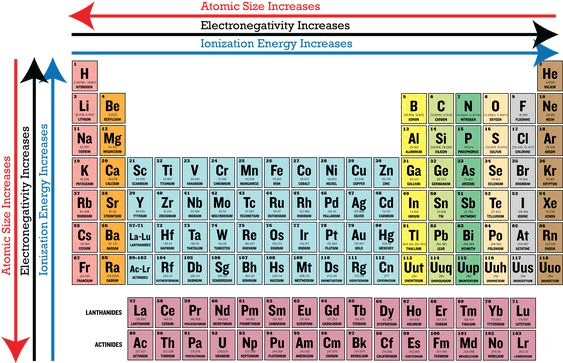Hence, the correct order of first ionization energy of Na, Mg, Al, and Si is 496, 737, 577, and 786 kJ mol–1 respectively.

Q.43. The increasing order of the atomic radii of the following elements is    
(I) C
(II) O
(III) F
(IV) Cl
(V) Br
(a) (II) < (III) < (IV) < (I) < (V)
(b) (IV) < (III) < (II) < (I) < (V)
(c) (III) < (II) < (I) < (IV) < (V)
(d) (I) < (II) < (III) < (IV) < (V)

Correct Answer is Option (c)

Moving left to right along a period, the size of the elements decreases and moving down the group, the size of the elements increases. Thus, Br is the biggest in size since it belongs to 4th period followed by Cl which belongs to 3rd period. Fluorine is the smallest element in the periodic table, and carbon comes before O in 2nd period. Hence, the correct increasing order of atomic radii is: (III), (II), (I), (IV), (V).

Q.44. B has a smaller first ionization enthalpy than Be. Consider the following statements:
(I) It is easier to remove 2p electron than 2s electron.
(II) 2p electron of B is more shielded from the nucleus by the inner core of electrons than the 2s electrons of Be.
(III) 2s electron has more penetration power than 2p electron.
(IV) Atomic radius of B is more than Be.
(Atomic number B = 5, Be = 4)
The correct statements are    
(a) (I), (II) and (IV)
(b) (II), (III) and (IV)
(c) (I), (II) and (III)
(d) (I), (III) and (IV)

Correct Answer is Option (c)

The electronic configuration of B (Z = 5) is 1s22s22pand Be (Z = 4) is 1s22s22p0. Thus, it is easier to remove an electron from B than Be. The 2p orbital of B is more shielded than 2s orbital of Be. s-orbital has more penetration power than p-orbital.

Q.45. The acidic, basic and amphoteric oxides, respectively, are    
(a) Na2O, SO3, Al2O3
(b) Cl2O, CaO, P4O10
(c) N2O3, Li2O, Al2O3
(d) MgO, Cl2O, Al2O3

Correct Answer is Option (c)

Non-metals of p-block elements form acidic oxide and metals of p-block elements form amphoteric oxide since they have properties in between metals and non-metals. Oxide of metals of s-block elements are basic in nature.
Hence,

Acidic oxide: N2O3
Basic oxide: Li2O
Amphoteric oxide: Al2O3

Q.46. If the magnetic moment of a dioxygen species is 1.73 BM, it may be   
(a)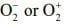(b)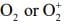(c)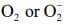(d)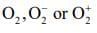Correct Answer is Option (a)

The molecular electronic configuration of the following species is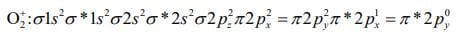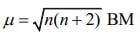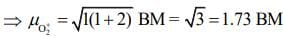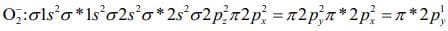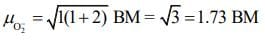Q.47. In general, the properties that decrease and increase down a group in the periodic table, respectively, are:    
(a) Atomic radius and electronegativity.
(b) Electron gain enthalpy and electronegativity.
(c) Electronegativity and atomic radius.
(d) Electronegativity and electron gain enthalpy.

Correct Answer is Option (c)

Generally, electronegativity decreases down the group as the size increases. This can also be formulated as:
Electronegativity = 1/ size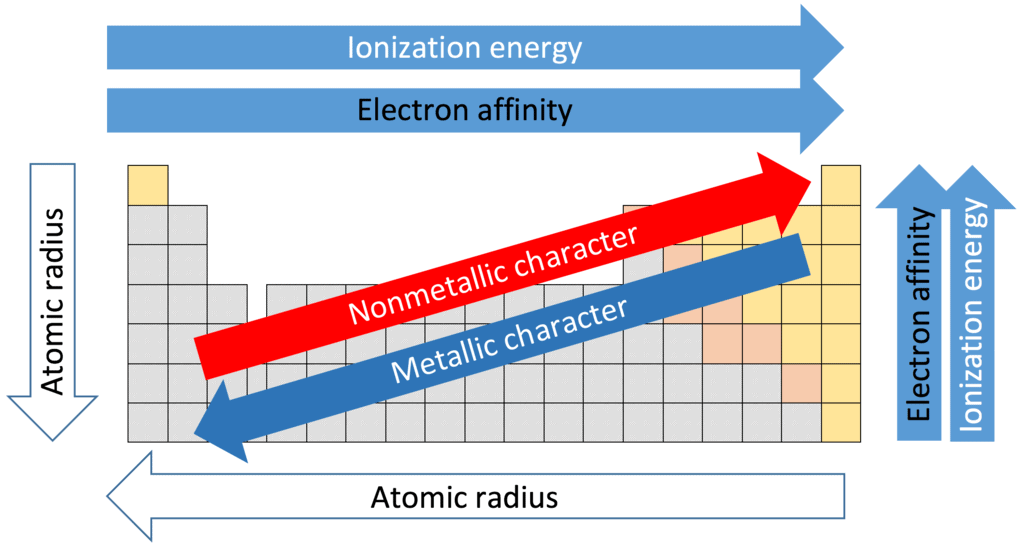Q.48. Aluminium is usually found in +3 oxidation state. In contrast, thallium exists in +1 and +3 oxidation states. This is due to: 
(a) Inert pair effect
(b) Diagonal relationship
(c) Lattice effect
(d) Lanthanoid contraction

Correct Answer is Option (a)

• Inert pair effect is the prominent character of the p-block element.
• In this, the high molecular weight element of the group show a lower oxidation state.
• This is because on going down the group, the shielding effect increases, but d-subshell and f-subshell show poor shielding effect.
• The high molecular weight members of p-block groups contain d-subshell and f-subshell. Because of this, they show a lower oxidation state.

SoDue to the inert pair effect, thallium exists in more than one oxidation state. Also, for thallium + 1 oxidation state is more stable than +3 oxidation state.

Q.49. When the first electron gain enthalpy(ΔegH) of oxygen is - 141 kJ/mol, its second electron gain enthalpy is:    
(a) A more negative value than the first
(b) Almost the same as that of the first
(c) Negative, but less negative than the first
(d) A positive value

Correct Answer is Option (d)

The second electron gain enthalpy of oxygen is positive as energy has to be added for the addition of another electron.

Q.50. The electronegativity of aluminium is similar to:    
(a) Carbon
(b) Beryllium
(c) Boron
(d) Lithium

Correct Answer is Option (b)

Be and Al shows diagonal relationship due to which these two elements have similar electronegativity.

Q.51. The 71st electron of an element X with an atomic number of 71 enters into the orbital:    
(a) 6p
(b) 4f
(c) 5d
(d) 6s

Correct Answer is Option (c)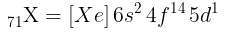∴ Orbital occupied by last e- is 5d.

Q.52. The correct order of the atomic radii of C, Cs, Al, and S is:   
(a) C < S < Al < Cs
(b) S < C < Cs < Al
(c) S < C < Al < Cs
(d) C < S < Cs < Al

Correct Answer is Option (a)

On going down the group, size increases while going from left to right in a period size decreases, so order is C < S < Al < Cs.

Q.53. The correct option with respect to the Pauling electronegativity values of the elements is:    
(a) Te > Se
(b) Ga<Ge
(c) Si < Al
(d) P > S

Correct Answer is Option (b)

Correct order of electronegativity values of the elements is
Si > Al; S > P; Se > Te; Ge > Ga.

Q.54. The relative stability of +1 oxidation state of group 13 elements follows the order:    
(a) Al < Ga < Tl < In
(b) Tl < In < Ga < Al
(c) Ga < Al < In < Tl
(d) Al < Ga < In < Tl

Correct Answer is Option (d)

Due to inert pair effect, the stability of +1 oxidation state increases down the group.
Thus, correct order of stability is Al < Ga < In < Tl

Q.55. The element with Z = 120 (not yet discovered) will be an/a:    
(a) Inner-transition metal
(b) Alkaline earth metal
(c) Alkali metal
(d) Transition metal

Correct Answer is Option (b)

Elements with Z = 120 will belong to alkaline earth metals.
Its electronic configuration may be represented as [Og] 8s2.

Q.66. The size of the iso-electronic species Cl-, Ar and Ca2+ is affected by:    
(a) Azimuthal quantum number of valence shell
(b) Electron-electron interaction in the outer orbitals
(c) Principal quantum number of valence shell
(d) Nuclear charge

Correct Answer is Option (d)

Iso-electronic species differ in size due to different effective nuclear charge.

Q.57. The IUPAC symbol for the element with atomic number 119 would be:    
(a) uue
(b) une
(c) unh
(d) uun

Correct Answer is Option (a)

Symbol for 1 is u and for 9 is e.
∴ UPAC symbol for 119 is uue.

Q.58. The correct statements among I to III regarding group 13 element oxides are,    
(I) Boron trioxide is acidic.
(II) Oxides of aluminium and gallium are amphoteric.
(III) Oxides of indium and thallium are basic.
(a) (I) and (II) only
(b) (I), (II) and (III)
(c) (I) and (III) only
(d) (II) and (III) only

Correct Answer is Option (b)
(I) B2O3 - Acidic Oxide
(II) Al2O3 & Ga2O3 - Amphoteric Oxide
(III) In2O3 & Tl2O - Basic Oxide

Q.59. In comparison to boron, beryllium has:    
(a) Lesser nuclear charge and lesser first ionisation enthalpy.
(b) Greater nuclear charge and lesser first ionisation enthalpy.
(c) Greater nuclear charge and greater first ionisation enthalpy.
(d) Lesser nuclear charge and greater first ionisation enthalpy.

Correct Answer is Option (d)

Nuclear charge : B > Be
Be = 1s2 2s2 (more stable)
B = 1s2 2s2p1
∴ Ionisation energy of Be is greater than B due to ns2 outer electronic configuration.

Q.60. For Na+, Mg2+, F- and O2-; the correct order of increasing ionic radii is:    
(a) Na
+ < Mg2+< F- < O2-
(b) Mg2 < Na+ < F- < O2-
(c) Mg2+ < O2- < Na+<  F-
(d) O2- < F- < Na+< Mg2+

Correct Answer is Option (b)

Isoelectronic series: Mg2+ < Na+ < F- < O2-
When negative charge increase, the radius of ion increases.

Q.61. The group having isoelectronic species is    
(a) O2, F, Na+, Mg2+
(b) O, F, Na, Mg+
(c) O2–, F, Na, Mg2+
(d) O, F, Na+, Mg2+

Correct Answer is Option (a)

Mg2+, Na+, O2– and F– all have 10 electrons each.

Q.62. Consider the following ionization enthalpies of two elements ‘A’ and ‘B’.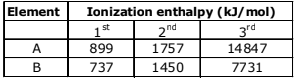Which of the following statements is correct?
(a) Both ‘A’ and ‘B’ belong to group-1 where ‘A’ comes below ‘B’.
(b) Both ‘A’ and ‘B’ belong to group-2 where ‘A’ comes below ‘B’.
(c) Both ‘A’ and ‘B’ belong to group-1 where ‘B’ comes below ‘A’.
(d) Both ‘A’ and ‘B’ belong to group-2 where ‘B’ comes below ‘A’.

Correct Answer is Option (d)

Generally, the ionization enthalpies or energy increases from left to right in a period and decreases from top to bottom in a group. Several factors such as atomic radius, nuclear charge, shielding effect are responsible for change of ionization enthalpies.
Here, Ist ionization enthalpy of A and B is greater than group I (Li 520 kJmol–1 to Cs 374 kJmol–1), which means element A and B belong to group –2 and all three given ionization enthalpy values are less for element B means B will come below A.

Q.63. The electronic configuration with the highest ionization enthalpy is:    
(a) [Ar] 3d10 4s4p3
(b) [Ne] 3s2 3p1
(c) [Ne] 3s2 3p3
(d) [Ne] 3s2 3p2

Correct Answer is Option (c)

S1< P1< S2< P2< P4< P3< P5< P6(IE order)

The document JEE Main Previous year questions (2016-22): Classification of Elements & Periodicity in Properties Notes | Study Chemistry for JEE - JEE is a part of the JEE Course Chemistry for JEE.
All you need of JEE at this link: JEE

## Chemistry for JEE

298 videos|517 docs|369 tests
 Use Code STAYHOME200 and get INR 200 additional OFF

## Chemistry for JEE

298 videos|517 docs|369 tests

Track your progress, build streaks, highlight & save important lessons and more!

,

,

,

,

,

,

,

,

,

,

,

,

,

,

,

,

,

,

,

,

,

;